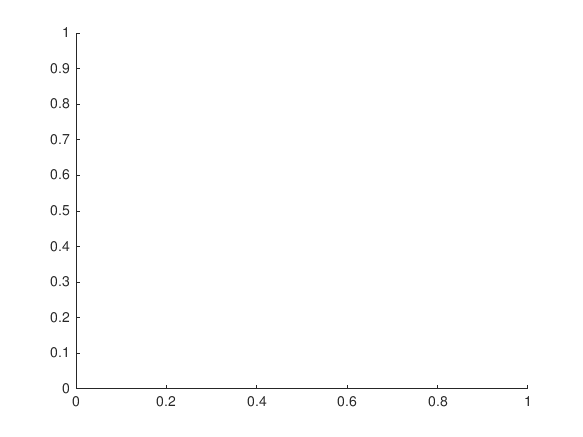Cody

# Problem 8048. Stress-Strain Properties - 1

Solution 1688372

Submitted on 7 Dec 2018
This solution is locked. To view this solution, you need to provide a solution of the same size or smaller.

### Test Suite

Test Status Code Input and Output
1   Pass
% The following properties are measured at room temperature and are tensile % in a single direction. Some materials, such as metals are generally % isotropic, whereas others, like composite are highly anisotropic % (different properties in different directions). Also, property values can % range depending on the material grade. Finally, thermal or environmental % changes can alter these properties, sometimes drastically.

2   Fail
S_y = 250e6; %Pa S_u = 400e6; %Pa e_y = 0.00125; e_u = 0.35; nu = 0.26; G = 79.3e9; %Pa E = 200e9; %Pa density = 7.85; %g/cm^3 sh_exp = 0.14; %strain-hardening exponent sh_coeff = 0.463; %strain-hardening coefficient assert(abs(stress_strain1(S_y,e_y)-E)<1e9)

Undefined variable "strain" or class "strain.GRD.GRD95.specimen1". Error in stress_strain1 (line 11) hold on, plot(strain.(type).(name).(spec)(:,2),stress.(type).(name).(spec)) Error in Test2 (line 11) assert(abs(stress_strain1(S_y,e_y)-E)<1e9)

``
3   Fail
S_y = 830e6; %Pa S_u = 900e6; %Pa e_y = 0.00728; e_u = 0.14; nu = 0.342; G = 44e9; %Pa E = 114e9; %Pa density = 4.51; %g/cm^3 sh_exp = 0.04; %strain-hardening exponent sh_coeff = 0.974; %strain-hardening coefficient assert(abs(stress_strain1(S_y,e_y)-E)<1e9)

Undefined variable "strain" or class "strain.GRD.GRD95.specimen1". Error in stress_strain1 (line 11) hold on, plot(strain.(type).(name).(spec)(:,2),stress.(type).(name).(spec)) Error in Test3 (line 11) assert(abs(stress_strain1(S_y,e_y)-E)<1e9)

4   Fail
S_y = 1172e6; %Pa S_u = 1407e6; %Pa e_y = 0.00563; e_u = 0.027; nu = 0.29; G = 11.6e9; %Pa E = 208e9; %Pa density = 8.19; %g/cm^3 sh_exp = 0.075; %strain-hardening exponent sh_coeff = 1.845; %strain-hardening coefficient assert(abs(stress_strain1(S_y,e_y)-E)<1e9)

Undefined variable "strain" or class "strain.GRD.GRD95.specimen1". Error in stress_strain1 (line 11) hold on, plot(strain.(type).(name).(spec)(:,2),stress.(type).(name).(spec)) Error in Test4 (line 11) assert(abs(stress_strain1(S_y,e_y)-E)<1e9)

5   Fail
S_y = 241e6; %Pa S_u = 300e6; %Pa e_y = 0.0035; e_u = 0.15; nu = 0.33; G = 26e9; %Pa E = 68.9e9; %Pa density = 2.7; %g/cm^3 sh_exp = 0.042; %strain-hardening exponent sh_coeff = 0.325; %strain-hardening coefficient assert(abs(stress_strain1(S_y,e_y)-E)<1e9)

Undefined variable "strain" or class "strain.GRD.GRD95.specimen1". Error in stress_strain1 (line 11) hold on, plot(strain.(type).(name).(spec)(:,2),stress.(type).(name).(spec)) Error in Test5 (line 11) assert(abs(stress_strain1(S_y,e_y)-E)<1e9)

6   Fail
S_y = 70e6; %Pa S_u = 220e6; %Pa e_y = 0.00054; e_u = 0.48; nu = 0.34; G = 48e9; %Pa E = 130e9; %Pa density = 8.92; %g/cm^3 sh_exp = 0.44; %strain-hardening exponent sh_coeff = 0.304; %strain-hardening coefficient 530MPa assert(abs(stress_strain1(S_y,e_y)-E)<1e9)

Undefined variable "strain" or class "strain.GRD.GRD95.specimen1". Error in stress_strain1 (line 11) hold on, plot(strain.(type).(name).(spec)(:,2),stress.(type).(name).(spec)) Error in Test6 (line 11) assert(abs(stress_strain1(S_y,e_y)-E)<1e9)

7   Fail
S_y = 317e6; %Pa S_u = 1130e6; %Pa e_y = 0.000685; e_u = 0.24; nu = 0.3; G = 178e9; %Pa E = 463e9; %Pa density = 21.02; %g/cm^3 sh_exp = 0.353; %strain-hardening exponent sh_coeff = 1.870; %strain-hardening coefficient assert(abs(stress_strain1(S_y,e_y)-E)<1e9)

Undefined variable "strain" or class "strain.GRD.GRD95.specimen1". Error in stress_strain1 (line 11) hold on, plot(strain.(type).(name).(spec)(:,2),stress.(type).(name).(spec)) Error in Test7 (line 11) assert(abs(stress_strain1(S_y,e_y)-E)<1e9)

8   Fail
S_y = 82e6; %Pa S_u = 82e6; %Pa e_y = 0.0265; e_u = 0.45; nu = 0.41; G = 2.8e9; %Pa E = 3.1e9; %Pa density = 1.14; %g/cm^3 assert(abs(stress_strain1(S_y,e_y)-E)<1e9)

Undefined variable "strain" or class "strain.GRD.GRD95.specimen1". Error in stress_strain1 (line 11) hold on, plot(strain.(type).(name).(spec)(:,2),stress.(type).(name).(spec)) Error in Test8 (line 9) assert(abs(stress_strain1(S_y,e_y)-E)<1e9)

9   Fail
S_y = 230e6; %Pa S_u = 230e6; %Pa e_y = 0.016; e_u = 0.016; nu = 0.35; G = 13.0e9; %Pa E = 14.5e9; %Pa density = 1.51; %g/cm^3 assert(abs(stress_strain1(S_y,e_y)-E)<1e9)

Undefined variable "strain" or class "strain.GRD.GRD95.specimen1". Error in stress_strain1 (line 11) hold on, plot(strain.(type).(name).(spec)(:,2),stress.(type).(name).(spec)) Error in Test9 (line 9) assert(abs(stress_strain1(S_y,e_y)-E)<1e9)

10   Fail
S_y = 1200e6; %Pa S_u = 1200e6; %Pa e_y = 0.001; e_u = 0.001; nu = 0.20; G = 478e9; %Pa E = 1200e9; %Pa density = 3.51; %g/cm^3 assert(abs(stress_strain1(S_y,e_y)-E)<1e9)

Undefined variable "strain" or class "strain.GRD.GRD95.specimen1". Error in stress_strain1 (line 11) hold on, plot(strain.(type).(name).(spec)(:,2),stress.(type).(name).(spec)) Error in Test10 (line 9) assert(abs(stress_strain1(S_y,e_y)-E)<1e9)